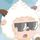cancel
Showing results for
Did you mean:New Member

## Add DATE filter to SUMX with multiple FILTER

Hello,

Warning! fairly new to DAX filtering in Power BI, but I am trying to do some calcultation on Azure Usage via the Concumption connector, I am trying to follow this guide for Excel https://learn.microsoft.com/en-us/azure/cost-management-billing/reservations/calculate-ea-reservatio...

'Usage details amortized' has more data than a calendar month, so I need a filter to only calculate calendar month, without date filter I have:

Test =

VAR UnitPrice =
SUMX(FILTER('Usage details amortized','Usage details amortized'[ReservationName] <> BLANK()&&'Usage details amortized'[ChargeType]="Usage" ),[UnitPrice])

VAR EffectivePrice1 =
SUMX(FILTER('Usage details amortized','Usage details amortized'[ReservationName] <> BLANK()&&'Usage details amortized'[ChargeType]="Usage" ),[EffectivePrice])

VAR Quantity =
SUM('Usage details amortized'[Quantity])

VAR EffectivePrice =
SUMX(FILTER('Usage details amortized','Usage details amortized'[ChargeType]="UnusedReservation"),[EffectivePrice])

VAR Quantity =
SUM('Usage details amortized'[Quantity])

RETURN
( UnitPrice - EffectivePrice1 - EffectivePrice) * Quantity

For date filter I was looking at using:
DATESBETWEEN('Usage details amortized'[Date],DATE(2022,10,1),DATE(2022,10,31))

If not using date filter is the use of SUMX the best way or should it be CALCULATE ?

1 ACCEPTED SOLUTIONCommunity Support

Hi @MKPDK ,

I suggest that you can use CALCULATE function, since you can add conditions without adding filter "FILTER( table" such as

Column = CALCULATE(SUM('actual table'[Actual value]),'actual table'[Id]=1).
Here are the differences about SUMX and CALCULATE.

SUMX is an iteration function in Power BI that works on a row-by-row calculation per the given expression or equation. This function considers each row at a time and applies the calculation.

When using the CALCULATE function, you can easily branch out to time intelligence calculations. CALCULATE function will change the context of your calculation in Power BI. It’s quite useful when you run some time intelligence calculation where you need to change the context.

You can reference the following document.

The CALCULATE Function In Power BI - DAX Tutorial - Microsoft Power BI Community

SUMX Power BI | How to use SUMX Function in Power BI? (with Example) (wallstreetmojo.com)

Best Regards,
Community Support Team _ xiaosun

If this post helps, then please consider Accept it as the solution to help the other members find it more quickly.Community Support

Hi @MKPDK ,

I suggest that you can use CALCULATE function, since you can add conditions without adding filter "FILTER( table" such as

Column = CALCULATE(SUM('actual table'[Actual value]),'actual table'[Id]=1).
Here are the differences about SUMX and CALCULATE.

SUMX is an iteration function in Power BI that works on a row-by-row calculation per the given expression or equation. This function considers each row at a time and applies the calculation.

When using the CALCULATE function, you can easily branch out to time intelligence calculations. CALCULATE function will change the context of your calculation in Power BI. It’s quite useful when you run some time intelligence calculation where you need to change the context.

You can reference the following document.

The CALCULATE Function In Power BI - DAX Tutorial - Microsoft Power BI Community

SUMX Power BI | How to use SUMX Function in Power BI? (with Example) (wallstreetmojo.com)

Best Regards,
Community Support Team _ xiaosun

If this post helps, then please consider Accept it as the solution to help the other members find it more quickly.